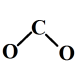# Parallel Analysis Criterion Simplified?

Am I missing something here? All of the macros I have seen for the parallel analysis criterion for factor analysis look pretty complicated, but, unless I am missing something, it is a simple deal.

The presumption is this:

There isn’t a number like a t-value or F-value to use to test if an eigenvalue is significant. However, it makes sense that the eigenvalue should be larger than if you factor analyzed a set of random data.

Random data is, well, random, so it’s possible you might have gotten a really large or really small eigenvalue the one time you analyzed the random data. So, what you want to do is analyze a set of random data with the same number of variables and the same number of observations a whole bunch of times.

Horn, back in 1965, was proposing that the eigenvalue should be higher than the average of when you analyzed a set of random data. Now, people are suggesting it should be higher than 95% of the time you analyzed random data (which kind of makes sense to me).

Either way, it seems simple. Here is what I did and it seems right so I am not clear why other macros I see are much more complicated. Please chime in if you see what I’m missing.

1. Randomly generate a set of random data with N variables and Y observations.
2. Keep the eigenvalues.
3. Repeat 500 times.
4. Combine the 500 datasets  (each will only have 1 record with N variables)
5. Find the 95th percentile

%macro para(numvars,numreps) ;
%DO k = 1 %TO 500 ;
data A;
array nums {&numvars} a1- a&numvars ;
do i = 1 to &numreps;
do j = 1 to &numvars ;
nums{j} = rand(“Normal”) ;
if j < 2 then nums{j} = round(100*nums{j}) ;
else nums{j} = round(nums{j}) ;
end ;
drop i j ;
output;
end;

proc factor data= a outstat = a&k  noprint;
var a1 – a&numvars ;
data a&k ;
set  a&k  ;
if trim(_type_) = “EIGENVAL” ;

%END ;
%mend ;

%para(30,1000) ;

data all ;
set a1-a500 ;

proc univariate data= all noprint ;
var a1 – a30 ;
output out = eigvals pctlpts =  95 pctlpre = pa1 – pa30;

*** You don’t need the transpose but I just find it easier to read ;
proc transpose data= eigvals out=eigsig ;
Title “95th Percentile of Eigenvalues ” ;
proc print data = eigsig ;
run ;

It runs fine and I have puzzled and puzzled over why a more complicated program would be necessary. I ran it 500 times with 1,000 observations and 30 variables and it took less than a minute on a remote desktop with 4GB RAM. Yes, I do see the possibility that if you had a much larger data set that you would want to optimize the speed in some way. Other than that, though, I can’t see why it needs to be any more complicated than this.

If you wanted to change the percentile, say, to 50, you would just change the 95 above. If you wanted to change the method from say, Principal Components Analysis (the default, with commonality of 1) to saying else, you could just do that in the PROC FACTOR step above.

The above assumes a normal distribution of your variables, but if that was not the case, you could change that in the RAND function above.

As I said, I am puzzled. Suggestions to my puzzlement welcome.

local_offerevent_note October 23, 2014

account_box

## 4 thoughts on “Parallel Analysis Criterion Simplified?”

•Abby Paden says:

I think a lot of the code out there in the ether is terribly optimized and unnecessarily complex. It’s as if some of the authors are trying to win an Obfuscated Code Challenge.

I will be using your PA program further into my degree.

•AnnMaria says:

Ha ha ha, you win my best comment of the week award!

•GM Jackson says:

In my opinion, finding a simpler, faster, more efficient way is always better. If something seems overly complicated, it’s usually because the creator of that complicated mess doesn’t want anyone to figure out how it works. It’s easier to monopolize a given task if you are the only one who knows how to do it. Once you simplify it, practically anyone can do it.

•AnnMaria says:

I’m the opposite – if practically anyone can do it then I’m freed up to find a new, more interesting challenge.

This site uses Akismet to reduce spam. Learn how your comment data is processed.